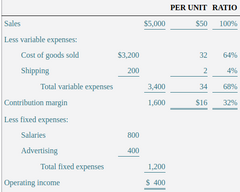Managerial Accounting Chapter 2.3 (Contribution Margin AnalysisDaphne Armenta
question

Operating Income Equations

Operating Income = Sales revenue – Total expenses Operating income = Sales revenue – Total variable expenses – Total fixed expenses Operating income = [(Sales price per – Variable cost per unit) x #of units sold] – fixed expenses
question

Contribution Margin

The difference between sales and variable costs—the amount that remains to cover fixed costs and provide a profit.
question

Contribution Margin & Contribution margin per unit formulas

Contribution margin = Sales revenue – Total variable expenses Contribution margin per unit = sales price per unit – Variable cost per unit
question

Operating Income formula using contribution margin per unit

[Contribution margin per unit x #of units sold] – Fixed Expenses
question

Contribution Margin Ratio

Contribution Margin ÷ Sales Revenue Contribution margin per unit ÷ Sales price per unit
question

Contribution Format Income Statement

An income statement that classifies costs by behavior
question

Contribution Format Income Statement vs GAAP Income Statement

Contribution Format Income statement helps managers predict the financial results of their decisions. It’s also based on cost behavior Meanwhile the GAAP Income statement doesn’t help to predict financial results, since it’s based on a cost function.
question

Contribution Format Income Statement Example

question

Variable Cost Ratio

Total Variable Cost ÷ Total Sales
question

The contribution margin is calculated as (a)Sales revenue − Cost of goods sold. (b)Sales revenue − Variable cost of goods sold. (c)Sales revenue − Total variable expenses. (d)Sales revenue − Total fixed expense

C.
question

The amount of net income presented on a functional income statement will be different from the amount of net income presented on a contribution format income statement. True or False?